• 可以输入长宽或半径来绘制平面图形，并可以计算他的周长与面积（通过接口实现），也可以选择绘制笔刷的颜色。Java图形界面实验可用
•AutoCAD
• 一.图形的绘制 1.绘制平面图形 1.1 绘制向量折线 x = [1, 3, 2 ,9, 0.5]; plot(x);...1.2 绘制平面曲线 %绘制 y = sin(x^2) x = -4 : 0.1 : 4; %x范围 y = sin(x.^2); %注意这里. 因为x相当于是矩阵
一.图形的绘制
对于下面的图形，已经给出了代码与详细注释，并没有给出具体画出的图形，建议初学者自己敲一下代码实现正确的图形显示
1.绘制平面图形
1.1 绘制向量折线图
x = [1, 3, 2 ,9, 0.5];
plot(x); %以元素该行序号为行坐标，以元素值为列下标，比如（1,1）（2,3）分别为第一个点与第二个点

1.2 绘制平面曲线图
%绘制 y = sin(x^2)
x = -4 : 0.1 : 4; %x范围
y = sin(x.^2); %注意这里. 因为x相当于是矩阵是对应元素相乘
plot(x, y);
grid on; %显示网格线 grid off即不显示网格线
gtext('sin(x^2)'); %可以图像结束之后在图形任意位置加上该函数括号里面的字符串% % x = 3cos t y = 2sin t
t = 0 : pi / 40 : 2 * pi;
x = 3 * cos(t);
y = 2 * sin(t);
plot(x, y);
plot(t, x, t ,y);
legend('3 * cos(t)', '2 * sin(t)'); %显示图注，默认在右上角
grid on;


hold on的用法
% %hold on ：可以让多个图像同时显示 hold off与之对应
x = -pi : pi / 40 : pi;
plot(x ,sin(x));
hold on; %这里如果不加，后面的曲线会覆盖前面的曲线
plot(x, cos(x));
figure用法
%一次运行出现多张图形，figure用法
figure(1); %表示出现图形一
% 图形颜色与画图线条的控制 详见下图
x = -pi : pi / 40 : pi;
plot(x ,sin(x), 'r.'); %'r.'代表红色以.组成的图形
hold on;
plot(x, cos(x), 'b-'); %'b-'代表蓝色以-组成的图形

figure(2); %表示出现图形二
x = -pi : pi / 40 : pi;
plot(x ,sin(x), 'r.'); %'r.'代表红色以.组成的图形
hold on;
plot(x, cos(x), 'b-'); %'b-'代表蓝色以-组成的图形
从上也可以看出在plot的第三个参数可以控制图形的线条与颜色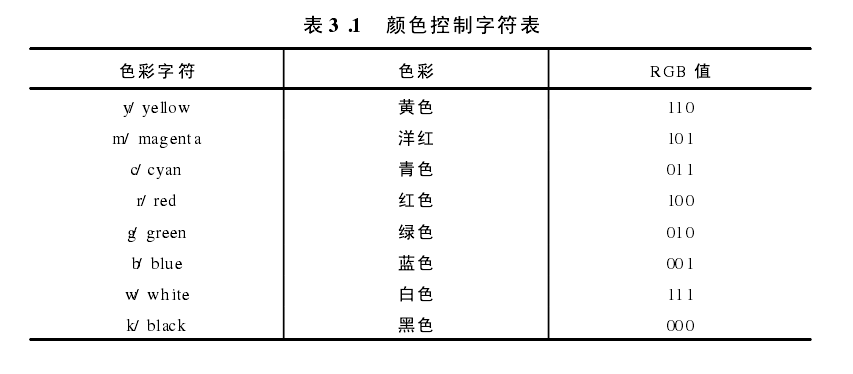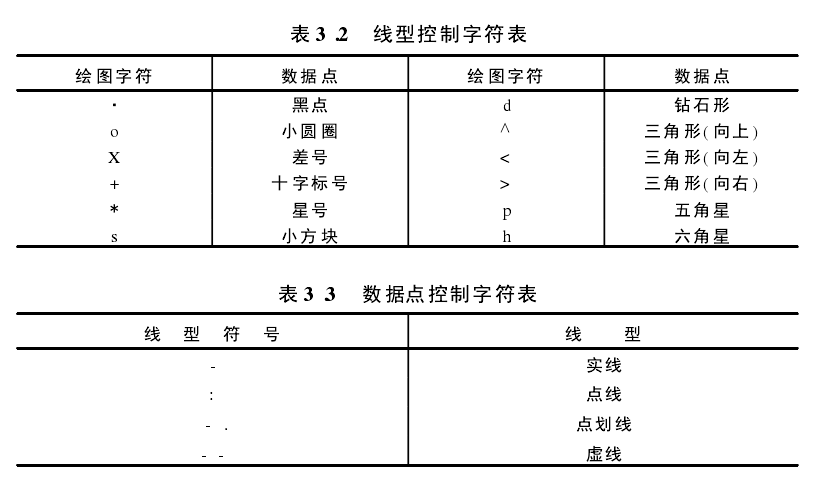坐标轴的名字与图形名字显示
x = -2 : 0.1 : 2;
y1 = cos(x);
y2 = x;
plot(x, y1, 'r-', x, y2, 'r-');
%先写plot 在写下面的图注信息，否则无效
title('cos(x)&x'); %在图形上面显示名字
xlabel('x'); %显示横坐标名字注意
ylabel('y');
legend('cos(x)', 'x');

一个图形多个子图的绘制
% 一个图形中多个子图的绘制
% subplot(x, y, z)：表示在x行y列的的矩阵的第z块绘制图形，块数以行为主开始数
clf; %清除所有图形界面
x = - 2: 0.2: 2;
y1 = x + sin(x) ;y2 = sin(x) ./ (x); %注意这里的./  .在前面
y3 = (1 + x) .^(1 ./x) ;
subplot(2,2,1) ,plot(x,y1,'m.' ) ,grid on, title('y = x + sinx') ;
%在2行2列的第一块绘制
subplot(2,2,2) ,plot(x,y2,'rp') ,grid on,title('y = sinx / x' ) ;
% 在2行2列的第二块绘制
subplot('position' , [0.2,0.05,0.6,0.45] ) , plot(x,y3) ,grid on;
% 在2行2列的第三第四块绘制图形，具体位置由 subplot('position' , [0.2,0.05,0.6,0.45] )
% 其中0.2表示距离左边界0.2；,0.05表示距离底部0.05； 0.6 0.45表示图形的具体宽和高
text(0, exp(1) ,'*'); %表示在坐标（0,exp(1)）c处用*标识

fplot与plot区别
figure(1);
x = -1 : 0.01 : 1;
y = sin(1 ./ x);
plot(x, y, 'r.');

figure(2);
hold on;
fplot('sin(1 ./x)', [-1, 1]); %自动选择图形点的步数，在点数密集的地方曲线越震荡或者密集


特殊图形的绘制

x = 1:5;
subplot(2,3,1) ,bar(x) , title('直方图' );
subplot(2,3,2) ,stairs(x) ,title('阶梯图');
subplot(2,3,3) ,stem(x,'rp') , title('火柴棍图');
subplot(2,3,4) ,pie(x) , title('饼图' );
subplot(2,3,5) ,pie3(x) ,title('三维饼图');
subplot(2,3,6) ,area(x) , title('面积图');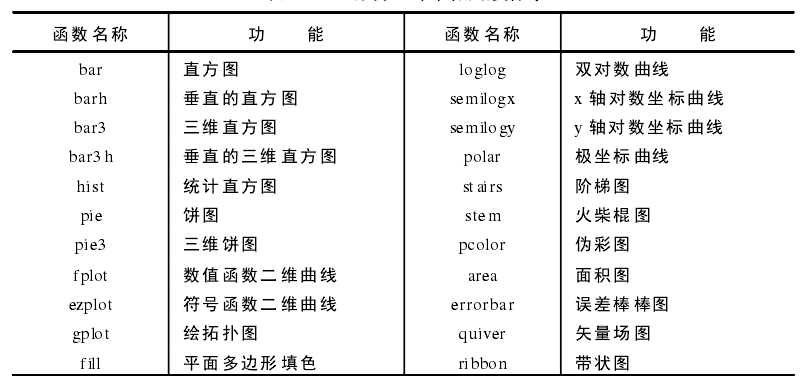展开全文matlab
CAD迷你 家装 是 室内设计 师经常用到的专业工具，对专业的设计人员来说，使用起来很方便基本一看就懂。但也有很多非专业的室内装修工作者使用，为方便新手使用本软件，小编这里用五步快速绘制室内CAD平面图来给大家讲解怎么用。项目：室内 CAD 平面图如下图所示：工具：CAD 迷你家装 V2.0步骤1：按尺寸绘制轴线使用CAD迷你家装图文教程画初始轴线，再用CAD迷你家装图文教程把其他轴线按尺寸画完。步骤 2：沿轴线绘制墙体此图墙宽度均为 240。使用绘制墙体，墙宽度选 240，沿轴线将墙画出步骤 3：在墙上自动插入窗窗定位：算出窗中心点位置，用绘制轴线和轴线偏移画出辅助的轴线；再使用标准窗，选择窗宽度，在正确位置插入窗。(C1 窗宽 1800，C2 窗宽 900)关闭轴线的图层检查一下：步骤 4：在墙上自动插入绘制门使用单开门，选择门的宽度，在墙体上添加门。(M0 门宽 900，M1 门宽 800，M2 门宽 700)步骤 5：添加构造柱、文字注释、尺寸标注使用插入柱子添加构造柱使用文字添加文字注释使用尺寸标注添加尺寸标注后续工作：在此户型图上布置家居、绘制厨卫、给排水、电 气、空调通风等 平面设计 图，请见后续教程。
展开全文• 基本架构import matplotlib.pyplot as pltplt.figure() ... plt.show()窗口plt.figure() plt.figure(num=None, figsize=None, dpi=None, facecolor=None, edgecolor=None, frameon=True, FigureClass=""" ...
基本架构

import matplotlib.pyplot as plt

plt.figure()
...
plt.show()

窗口

plt.figure()

plt.figure(num=None, figsize=None, dpi=None, facecolor=None, edgecolor=None, frameon=True, FigureClass=

"""
示例：
"""

# 编号为3；大小为(8, 5).
plt.figure(num=3, figsize=(10, 5))

绘图

plt.plot()

画线。

"""
示例：
"""

import numpy as np
# 使用np.linspace定义x：范围是(-3,3);个数是50.
x = np.linspace(-3, 3, 50)

# 仿真一维数据组(x ,y1)表示曲线1.
y1 = 2*x + 1
# 仿真一维数据组(x ,y2)表示曲线2.
y2 = x**2

plt.plot(x, y1)
# 曲线的颜色属性(color)为红色;曲线的宽度(linewidth)为1.0；曲线的类型(linestyle)为虚线. 使用plt.show显示图像.
plt.plot(x, y2, color='red', linewidth=1.0, linestyle='--')

坐标轴

plt.xlim() / plt.ylim()

调整 坐标轴 范围。

"""
示例：
"""

# 使用plt.xlim设置x坐标轴范围：(-1, 2)；
plt.xlim((-1, 2))
# 使用plt.ylim设置y坐标轴范围：(-2, 3)；
plt.ylim((-2, 3))

plt.xlabel() / plt.ylabel()

调整 坐标轴 名称。

"""
示例：
"""

# 使用plt.xlabel设置x坐标轴名称：’I am x’；
plt.xlabel('I am x')
# 使用plt.ylabel设置y坐标轴名称：’I am y’；
plt.ylabel('I am y')

plt.xticks / plt.yticks()

调整 坐标轴 刻度间隔。

"""
示例：
"""

# 使用plt.xlim设置x坐标轴范围：(-1, 2)；
new_ticks = np.linspace(-1, 2, 5)
plt.xticks(new_ticks)
# 使用plt.ylim设置y坐标轴范围：(-2, 3)；
plt.ylim((-2, 3))

Ref：

Pyplot tutorial
莫烦Python - Matplotlib
matplotlib 绘图可视化知识点整理
Matplotlib 教程

展开全文matplot python
• 1.平面图形的绘制 思路: 首先自己想象一个形状 3D坐标系统是(左上角-1,1,0 右下角1,-1,0),可以根据手机屏幕宽高调整坐标，防止图形旋转时的拉伸。 然后根据安卓手机的坐标系统确定每个顶点的坐标。 所有的坐标...
1.平面图形的绘制

思路:

首先自己想象一个形状
3D坐标系统是(左上角-1,1,0  右下角1,-1,0),可以根据手机屏幕宽高调整坐标，防止图形旋转时的拉伸。
然后根据安卓手机的坐标系统确定每个顶点的坐标。
所有的坐标都是（x,y,z）形式并且存于一个float[] 。
由于OpenGLES是用单个三角形组成矩形等其它图形。且每3个点以逆时针方向形成的是一个正面。
所以需要一个byte[] 来存储这些顶点，并且按照顺序排列。这样就知道有哪些点参与了绘制，并知道这些点是按照何种顺序进行绘制三角形的，然后三角形组成其它图形。
有了顶点数组float[] 和 面数组byte[] ,但是不能直接使用,需要转化才能在代码中使用
float[] 转化为 FloatBuffer
byte[] 转化为 ByteBuffer
暂时到这里就结束了，可以直接在代码中使用并绘制图形了。之后还会多一个纹理绘制，之后再讲。

2.API介绍

一般实现Renderer接口，重写三个方法，onSurfaceCreated()   onSurfaceChanged()   onDrawFrame()

onSurfaceCreated()中一般做一些初始化设置

@Override
public void onSurfaceCreated(GL10 gl, EGLConfig config) {
//关闭抗抖动
gl.glDisable(GL10.GL_DITHER);
//修正系统透视
gl.glHint(GL10.GL_PERSPECTIVE_CORRECTION_HINT , GL10.GL_FASTEST);
//设置阴影平滑模式
//启用深度测试 记录Z轴深度
gl.glEnable(GL10.GL_DEPTH_TEST);
//设置深度测试的类型
gl.glDepthFunc(GL10.GL_LEQUAL);
}

onSurfaceChanged() 常用来初始化3D场景

@Override
public void onSurfaceChanged(GL10 gl, int width, int height) {
//设置3D窗口的大小及位置
gl.glViewport(0 , 0 , width , height );
//将矩阵模式设置为投影矩阵
gl.glMatrixMode(GL10.GL_PROJECTION);
//初始化单位矩阵
//计算透视窗宽高比
float ratio = (float)width/height;
//设置透视视窗的空间大小 默认为 -1,1,-1,1,-1,1
//gl.glFrustumf(-ratio , ratio , -1 , 1  ,1 , 10);
}

onDrawFrame() 进行绘制

 @Override
public void onDrawFrame(GL10 gl) {
//清除屏幕缓存和深度缓存
gl.glClear(GL10.GL_COLOR_BUFFER_BIT | GL10.GL_DEPTH_BUFFER_BIT);
//启用顶点坐标数据
gl.glEnableClientState(GL10.GL_VERTEX_ARRAY);
//设置当前矩形堆栈为模型堆栈
gl.glMatrixMode(GL10.GL_MODELVIEW);

//重置当前的模型视图矩阵
//如果需要用颜色填充平面,还需要禁用顶点颜色数据
gl.glColor4f(0.2f , 1.0f , 0.2f , 0.0f);
gl.glDisableClientState(GL10.GL_COLOR_ARRAY);
//绕y轴旋转
gl.glRotatef(roate , 0f , 1f , 0f);
//设置顶点位置数据vertextBuffer
gl.glVertexPointer(3 , GL10.GL_FLOAT , 0 , vertextBuffer);
//绘制面数据faceBuffer
gl.glDrawElements(GL10.GL_TRIANGLES, faceBuffer.remaining() , GL10.GL_UNSIGNED_BYTE , faceBuffer);

//停止绘制
gl.glFinish();
//停用坐标数据
gl.glDisableClientState(GL10.GL_VERTEX_ARRAY);

roate+=1;
if (roate == 360){
roate = 0;
}
}

接下来是一个矩形的绘制：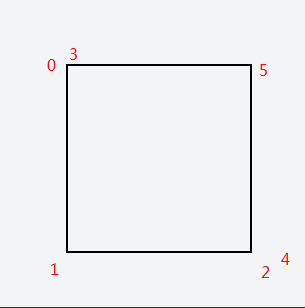我们先用0，1，2三个顶点绘制第一个三角形，然后用3，4，5三个顶点绘制第二个三角形。都是逆时针顺序，显示的就是正面。然后两个三角形组成矩形。

有些点在空间上是重复的，在设置顶点数组的时候，可以只设置四个点，然后在面数组中你重复利用4个点就行了，但是为了之后的纹理绘制不出错，因为重复利用顶点经过试验导致发生了错误，所以2个三角形，6个点，每个点都有值,不重复利用顶点，而不是简单的给4个点。

这样你的顶点数组内容应该是：

float[]={

x0 , y0 , z0 ,

x1 , y1 , z1 ,

x2 , y2 , z2 ,

x3 , y3 , z3 ,

x4 , y4 , z4 ,

x5 , y5 , z5

};

面数组的内容应该是：byte[] = {0,1,2,3,4,5}

3.代码：

public class MainActivity extends AppCompatActivity {

@Override
protected void onCreate(Bundle savedInstanceState) {
super.onCreate(savedInstanceState);

GLSurfaceView view = new GLSurfaceView(this);

setContentView(view);

}
}

public class OtherShader implements GLSurfaceView.Renderer{
FloatBuffer vertextBuffer;
ByteBuffer faceBuffer;
private float roate;
Context context;

this.context = context;
//转化
vertextBuffer = floatArray2Buffer(vertex);
faceBuffer = ByteBuffer.wrap(face);
}
//顶点
float[] vertex = new float[]{
-0.5f , 0.5f , 0f ,
-0.5f , -0.5f , 0f ,
0.5f , -0.5f , 0f ,
-0.5f , 0.5f , 0f ,
0.5f , -0.5f , 0f ,
0.5f , 0.5f , 0f
};
//面
//两个三角形组成一个矩形
byte[] face = new byte[]{
0,1,2, //第0，1，2个顶点组成一个三角形
3,4,5
};

//将顶点颜色数组转换为IntBuffer,是OpenGl ES所需要的。也可以不设置顶点颜色数组int[]
private IntBuffer intArray2Buffer(int[] rect1color) {
IntBuffer intBuffer;
//不用该方法得到IntBuffer,因为Android平台限制，Buffer必须为native Buffer,所以要通过allocateDirect()创建
//并且该Buffer必须是排序的，所以要order()方法进行排序
//intBuffer = IntBuffer.wrap(rect1color);
//因为一个int=4字节
ByteBuffer bb = ByteBuffer.allocateDirect(rect1color.length * 4);
bb.order(ByteOrder.nativeOrder());
intBuffer = bb.asIntBuffer();
intBuffer.put(rect1color);
intBuffer.position(0); //移到第一个点的数据
return intBuffer;
}

//将顶点位置数组转换为FloatBuffer,是OpenGl ES所需要的
private FloatBuffer floatArray2Buffer(float[] rect1) {
FloatBuffer floatBuffer;
//不用该方法得到FloatBuffer,因为Android平台限制，Buffer必须为native Buffer,所以要通过allocateDirect()创建
//并且该Buffer必须是排序的，所以要order()方法进行排序
//floatBuffer = FloatBuffer.wrap(rect1);
//因为一个int=4字节
ByteBuffer bb = ByteBuffer.allocateDirect(rect1.length * 4);
bb.order(ByteOrder.nativeOrder());
floatBuffer = bb.asFloatBuffer();
floatBuffer.put(rect1);
floatBuffer.position(0); //移到第一个点的数据
return floatBuffer;
}

@Override
public void onSurfaceCreated(GL10 gl, EGLConfig config) {
//关闭抗抖动
gl.glDisable(GL10.GL_DITHER);
//修正系统透视
gl.glHint(GL10.GL_PERSPECTIVE_CORRECTION_HINT , GL10.GL_FASTEST);
//设置阴影平滑模式
//启用深度测试 记录Z轴深度
gl.glEnable(GL10.GL_DEPTH_TEST);
//设置深度测试的类型
gl.glDepthFunc(GL10.GL_LEQUAL);

}

@Override
public void onSurfaceChanged(GL10 gl, int width, int height) {
//设置3D窗口的大小及位置
gl.glViewport(0 , 0 , width , height );
//将矩阵模式设置为投影矩阵
gl.glMatrixMode(GL10.GL_PROJECTION);
//初始化单位矩阵
//计算透视窗宽高比
float ratio = (float)width/height;
//设置透视视窗的空间大小 默认为 -1,1,-1,1,-1,1
//gl.glFrustumf(-ratio , ratio , -1 , 1  ,1 , 10); //防止图形旋转拉伸，可在这里调整
}

@Override
public void onDrawFrame(GL10 gl) {
//清除屏幕缓存和深度缓存
gl.glClear(GL10.GL_COLOR_BUFFER_BIT | GL10.GL_DEPTH_BUFFER_BIT);
//启用顶点坐标数据
gl.glEnableClientState(GL10.GL_VERTEX_ARRAY);
//设置当前矩形堆栈为模型堆栈
gl.glMatrixMode(GL10.GL_MODELVIEW);

//重置当前的模型视图矩阵
//如果需要用颜色填充平面,还需要禁用顶点颜色数据
gl.glColor4f(0.2f , 1.0f , 0.2f , 0.0f);
gl.glDisableClientState(GL10.GL_COLOR_ARRAY);
//绕y轴旋转
gl.glRotatef(roate , 0f , 1f , 0f);
//设置顶点位置数据
gl.glVertexPointer(3 , GL10.GL_FLOAT , 0 , vertextBuffer);
//绘制面
gl.glDrawElements(GL10.GL_TRIANGLES, faceBuffer.remaining() , GL10.GL_UNSIGNED_BYTE , faceBuffer);

//停止绘制
gl.glFinish();
//停用坐标数据
gl.glDisableClientState(GL10.GL_VERTEX_ARRAY);

roate+=1;
if (roate == 360){
roate = 0;
}
}

}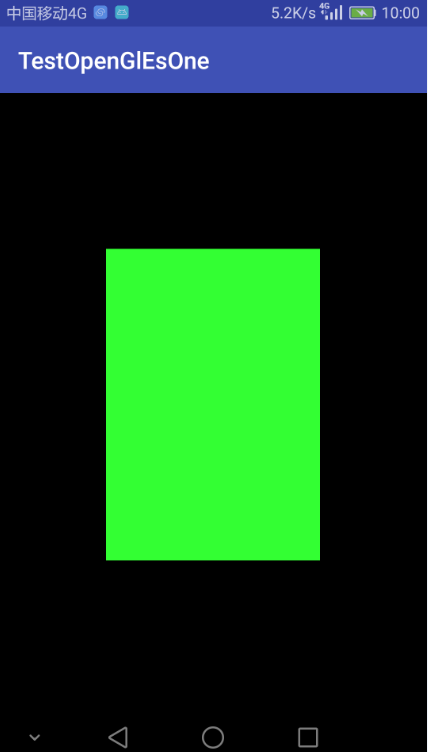之后会讲给这个平面进行纹理贴图.。


展开全文•  数组类型的地图  ...行编号=索引值/每一行的块个数；...列编号=索引值%每一行的块个数；...小块贴图坐标的公式 ： ...左上点X坐标=行编号×块的宽度;...左上点Y坐标=列编号×块的高度;...地图宽=列数×winapi delete null system
• <br />转自：...本节将介绍MATLAB基本xy平面及xyz空间的各项绘图命令，包含一维曲线及二维曲面的绘制、列印及存档。  <br />plot是绘制一维曲线的基本函数，但在使用matlab plot function
• 摘要： 　HTML5规范已经完成了，互联网上已经有数... 历史： ...它自己没有行为，但却把一个绘图 API 展现给客户端 JavaScript 以使脚本能够把想绘制的东西都绘制到一块画布上。canvas标记由 Apple 在 Safari...
• 但是在使用计算机绘图软件绘制平面图前，首先你必须先读懂室内平面图画法、平面的配置原则与常见的平面图种类。如何看懂室内平面图？从2基础概念开始着手1.基础空间概念想要画出一张人人都能...
• 绘制以下系统的相平面图(d^2y/dt^2)+2Jw(dy/dt)+w^2=0 对于不同的 w & J 值matlab
• 这是一个wpf语言绘制的2d图形！ 是c#做后台代码的！
• 教大家用visio可以绘制家居平面图，简单的说明，大家一看就会了。
• 利用Excel自选图形制作室内平面图.rar,室内平面结构图反映了居室的布局和各房间的功能、面积，同时还决定了门、窗的位置。在没有专业的室内设计软件时，使用Excel也能快速绘制出排列的室内平面图Excel应用
• Apex Sketch是一款好用的平面图绘制软件，它可以让用户更轻松地绘制平面图，而且还支持传统的键盘和鼠标输入以及带有Leica®DISTO™激光测量仪的有源笔，触摸和蓝牙。操作也很简单，你只需点击几下鼠标，您只需绘制...
• MATLAB相图程序 Eg1: clc;clear;close; [x,y]=meshgrid(linspace(-6,6)); %定义[x,y]网络采样点的范围 ...title('极限环的相平面图') %定义标题范围 xlabel('x','Color',[0.6 0.5 0.4]);ylabel('y','Cmatlab 算法
•绘图工具
• 使用visio绘图软件绘制平面图纸，标注了信息点等。
• MapGis CAD 气象 地质 水文 制图 地图 测绘
• 甚至创造性的仅仅使用Photoshop中的一些功能，就完成了逼真生动的手绘彩色平面图绘制。这套教程基本涵盖了彩色平面绘制的所有常用技巧，希望可以帮助你举一反三，创造出属于自己的独特表现风格。第一章 P......